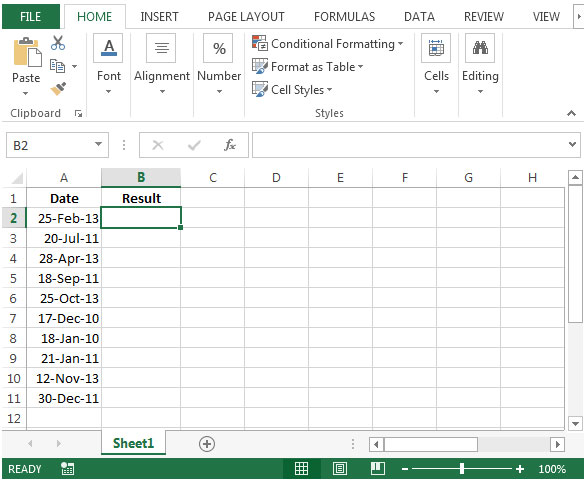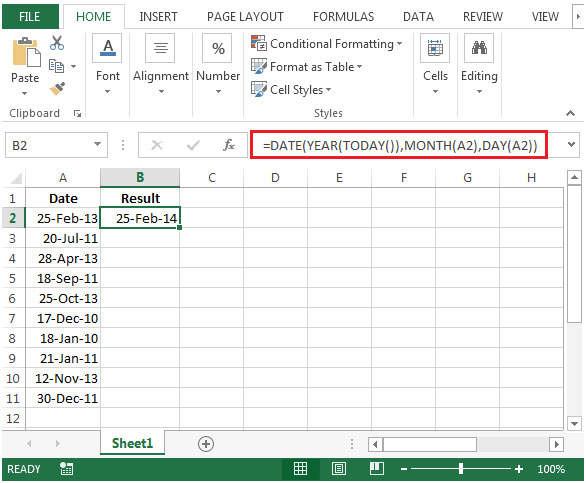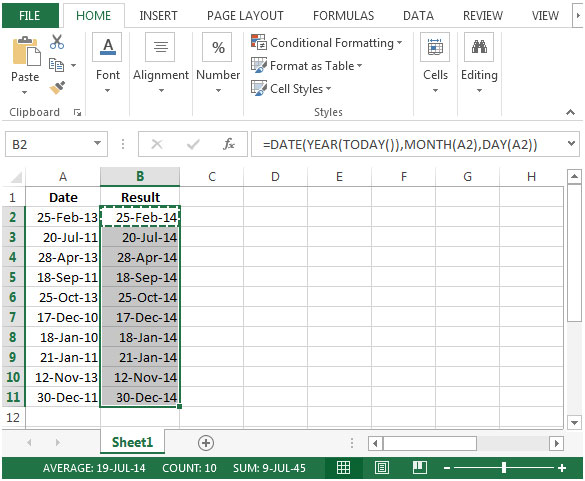# Converting Older Dates To The Current Year In Microsoft Excel 2010

In this article, we will learn how to change color numbers based on the value result in Microsoft Excel 2010.

You may have found a scenario in which you are required to convert the older dates to the same date of the current year. You can create a formula that will return the output as required.

You can use a combination of DATE, YEAR, TODAY, MONTH & DAY functions to convert to date of the current year from older dates.

DATE: Returns the number that represents the date in Microsoft Excel date-time code.

Syntax: =DATE(year,month,day)

year: The year argument can include one to four digits. By default, Excel uses the 1900 date system.

month: The second argument representing the month of the year from 1 to 12 (January to December)

day: The third argument representing the day of the month from 1 to 31.

YEAR: Returns the year of a date, an integer in the range 1900 - 9999

Syntax: =YEAR(serial_number)

serial_number: The date of the year you want to find.

TODAY: Returns the current date formatted as a date

Syntax: =TODAY()

TODAY function does not have any argument.

MONTH: This function returns the month (January to December as 1 to 12) of a date.

Syntax: =MONTH(serial_number)

serial_number: It refers to the date of the month that you are trying to find.

DAY: Returns the day of the month, a number from 1 to 31

Syntax: =DAY(serial_number)

serial_number: It refers to the day of the month that you are trying to find.

Let us take an example:

• We have some random Dates in column A
• In column B, we want to find the result. A formula that will take the value from corresponding cell & convert it to the same date of the current year.• In cell B2, the formula would be =DATE(YEAR(TODAY()),MONTH(A2),DAY(A2))• The Year will be calculated as current year by taking reference from Today function i.e. the function would be YEAR(TODAY()). We will get 2014
• Month & Day are being calculated by taking reference from date in column A. After that, we will get Month as Feb& Day as 25
• Copying the formula in cell B2 to range B3:B11, we will get the desired result.1.